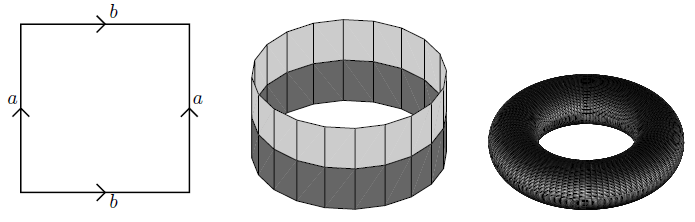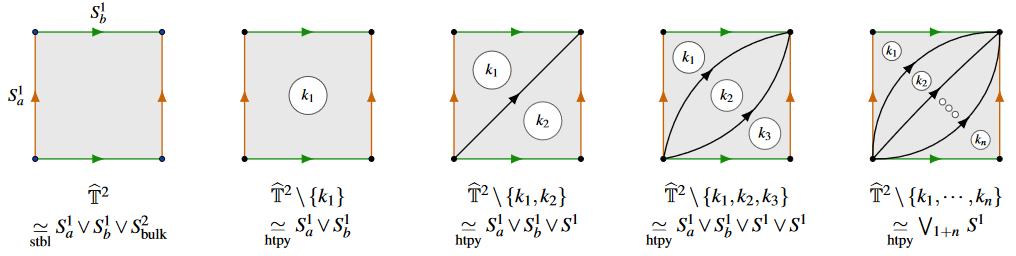Contents

# Contents

## Definition

### In topology and differential geometry

The torus is the manifold (a smooth manifold, hence in particular also a topological manifold) obtained as the quotient

$T \coloneqq \mathbb{R}^2 / \mathbb{Z}^2$

of the Cartesian plane, regarded as an abelian group, by the subgroup of pairs of integers.

As a topological space this is the quotient topological space obtained from the square by identifying opposite sides:graphics grabbed from Lawson 03

More generally, for $n \in \mathbb{N}$ any natural number, the $n$-torus is

$T \coloneqq \mathbb{R}^n / \mathbb{Z}^n \,.$

For $n = 1$ this is the circle.

In this fashion each torus canonically carries the structure of an abelian group, in fact of an abelian Lie group. Notice that regarded as a group the torus carries a base point (the neutral element).

### In algebraic geometry

According to SGA3, for $X$ a base scheme then a 1-dimensional torus (in the sense of tori-as-groups) over it is a group scheme over $X$ which becomes isomorphic to the multiplicative group over $X$ after a faithfully flat group extension.

In (Lawson-Naumann 12, def. A.1) this is called “a form of” the multiplicative group over $X$.

By (Lawson-Naumann 12, prop. A.4) the moduli stack of 1-dimensional tori $\mathcal{M}_{1dtori}$ in this sense is equivalent to the delooping of the group of order two:

$\mathcal{M}_{1dtor} \simeq \mathbf{B}\mathbb{Z}/2\mathbb{Z} \,.$

The single nontrival automorphism of any 1-dimensional toris here is that induced by the canonical automorphism of the multiplicative group

$Aut(\mathbb{G}_m) \simeq \mathbb{Z}/2\mathbb{Z}$

which is the inversion involution (given by sending any element to its inverse element).

### As a homotopy type

As a homotopy type the torus is the product of two copies of the circle.

In homotopy type theory the torus can be formalized as the higher inductive type generated by a point base, two paths, $p$ and $q$, from base to itself, and an element $t$ of $p\cdot q = q \cdot p$. It has been formally shown (Sojakova15) that this type is equivalent to the product of two circles. For a treatment in cubical type theory, see (Licata-Brunierie).

$\,$

The stable homotopy type of the torus is the wedge sum of 2 circles and one 2-sphere (eg. Freed & Moore 13, Thm. 11.8):(graphics from SS 22)

## Properties

• Terry Lawson, Topology: A Geometric Approach, Oxford University Press (2003) (pdf)

The moduli stack of 1-dimensional tori in algebraic geometry is discussed (as the cusp point inside the moduli stack of elliptic curves) in

Discussion of tori the homotopy type of the torus in homotopy type theory is in

On 2-group-extensions of torus groups:

On the stable homotopy type of the torus: